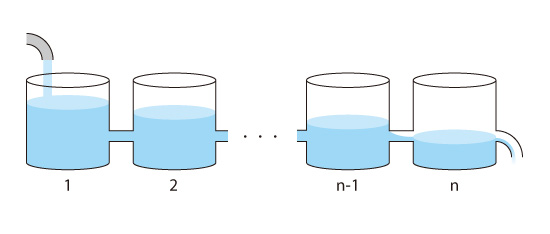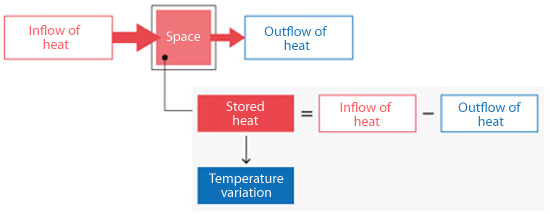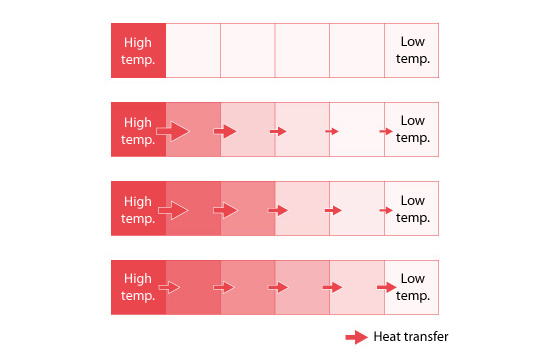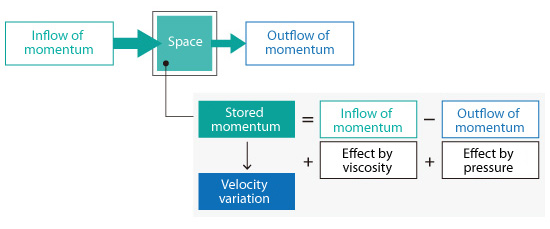# Basic Course of Thermo-Fluid Analysis 13: Chapter 5 Basics of thermo-fluid analyses - 5.2 Finite volume method

#### 5.2 Finite volume method

The finite volume method (FVM) is a method for estimating the amount of a physical quantity stored in a space based on the difference between the amount of inflow and outflow to the space.

Consider the situation where water is poured into a container as shown in Figure 5.4. The amount of water (variation) that accumulates in the container over a certain period is obtained from the difference between the amount of inflow and outflow. When the amount of accumulated water is determined, the change in the water level can be calculated knowing the dimensions of the container. This change in water level is an example of how a physical quantity is calculated using the finite volume method.Figure 5.4: Concept of finite volume method

In an actual analysis, the analysis target is divided into many small containers or spaces. The containers are connected as shown in Figure 5.5.Figure 5.5: Analysis target is divided into multiple spaces

The amount of outflow from one container is equal to the amount of water flowing into the next container.

In the above example,
Outflow from 1 = Inflow to 2
2 Outflow from 2 = Inflow to 3
:
:
n-Outflow from n-1 = Inflow to n

Now, let's take a more concrete example. To obtain the temperature from the energy conservation equation, consider the amount of heat flowing into a space due to thermal conduction and heat convection, and the amount of heat flowing out from the space. The goal is to obtain the temperature variation (change in temperature) from the amount of stored heat.Figure 5.6: Inflow and outflow of heat

The inflow and outflow of heat are calculated for each space and the amount of stored heat for each space is calculated from these values. Once the amount of stored heat is known for each space, temperature in each space can be calculated.

Figure 5.7 is used to conceptually illustrate the calculation process for achieving the steady state temperature of a part that is heated at one end. The left end is at a high temperature and the right end is at a low temperature. Initially the temperatures between the two ends are unknown. Starting from the left end, the temperature gradually rises in the adjacent spaces as heat flows from a space of higher temperature to that of lower temperature. The amount of heat flowing into each space is greater than the amount of heat flowing out just after the start because the temperature difference between the first two cells on the left is higher than the difference between any of the other adjacent cells. After a sufficiently long time has passed, the amount of heat flowing into and out from each space is balanced as shown in the bottom of the figure* . Here, the temperature does not change and the steady state condition is achieved.

* The state corresponds to the state where the amounts of inflow and outflow in all the containers are balanced (water level does not change) in Figure 5.5.Figure 5.7: Heat transfer and temperature distribution

This basic concept also applies to flows. The velocity change in each space is obtained by considering both the momenta flowing in and out of the space but also viscosity and pressure effects.Figure 5.8: Inflow and outflow of momentum

Note that there is no governing equation to solve for pressure. To obtain pressure, other methods are used. For example, the fluid velocity can be calculated using an estimate of the pressure. Then the pressure is corrected such that the obtained velocity satisfies the equation of continuity.